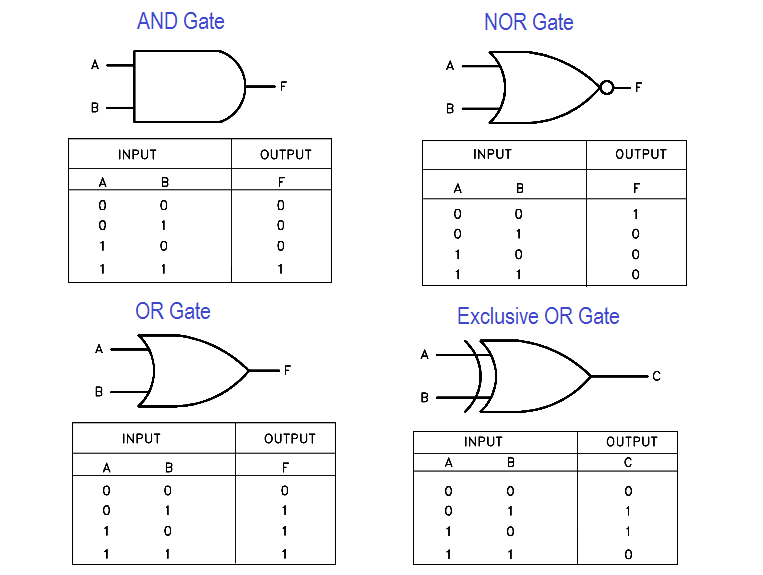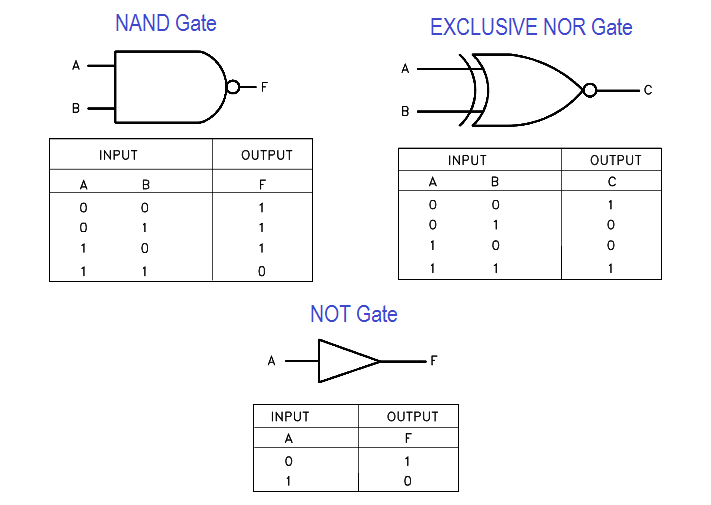# Logic Gates and Truth tables

Truth tables offer a simple and easy to understand tool that can be used to determine the output of any logic gate or circuit for all input combinations.

#### Truth Tables

When a logic gate has only two inputs, or the logic circuit to be analyzed has only one or two gates, it is fairly easy to remember how a specific gate responds and determine the output of the gate or circuit. But as the number of inputs and/or the complexity of the circuit grows, it becomes more difficult to determine the output of the gate or circuit.

Truth tables, as illustrated in Figure 11, are tools designed to help solve this problem.

A truth table has a column for the input of each gate and column for the output of each gate. The number of rows needed is based on the number of inputs, so that every combination of input signal is listed (mathematically the number of rows is 2 , where n = number of inputs).

In truth tables, the on and off status of the n inputs and outputs is represented using 0s and 1s. As previously stated 0 = off and 1 = on. Figure 11 lists truth tables for the seven basic logic gates. Compare each gate’s truth table with its definition given earlier in this module, and verify for yourself that they are stating the same thing.Figure 11 Truth Tables